# Chapter 12: Java GUI Programming

• Java GUI 技术
• JavaFX 基础知识
• 带有 JavaFX 的 HelloWorld
• 控制元件
• 图表
• 应用 CSS
• 使用 FXML
• 嵌入 HTML
• 播放媒体
• 添加效果

# Technical requirements

• 装有 Microsoft Windows、Apple macOS 或 Linux 操作系统的计算机
• Java SE 版本 17 或更高版本
• 您选择的 IDE 或代码编辑器

# Java GUI technologies

Java Foundation Classes (JFC) 的名称可能是一个来源 非常混乱。它暗示 Java 基础的类，而事实上，JFC 只包括与 GUI 相关的类和接口。准确地说，JFC 是三个框架的集合：Abstract Window Toolkit (AWT)、Swing 和 Java 2D。

JFC 是 Java 类库 (JCL) 的 的一部分，虽然名称JFC 于 1997 年才成立，而 AWT 从一开始就是 JCL 的一部分。当时，Netscape 开发了一个名为 Internet Foundation Classes (IFC) 的 GUI 库，以及 Microsoft 为 GUI 创建了应用程序基础类 (AFC)发展，也是。因此，当 Sun Microsystems 和 Netscape 决定组建一个新的 GUI 库时，他们继承了 Foundation 这个词并创建了 JFC。 Swing 框架从 AWT 接管了 Java GUI 编程，并成功使用了近二十年。

Java 8 中的 JCL 中添加了一个新的 GUI 编程工具包 JavaFX。它在 Java 11 中从 JCL 中删除，从那时起，作为 Gluon 公司支持的开源项目，作为 JDK 之外的可下载模块. JavaFX 使用与 AWT 和 Swing 不同的 GUI 编程方法。它提供了更一致的 和更简单的设计，并且很有可能成为获胜的 Java GUI 编程工具包。

# JavaFX fundamentals

JavaFX 中包含所有其他组件的顶级容器由 `javafx.stage.Stage` 类表示。因此，您可以说，在 JavaFX 应用程序中，一切都发生在 stage 上。从用户的角度来看，它是一个显示区域或窗口，所有控件和组件都在其中执行其操作（就像剧院中的演员）。而且，与剧院中的演员类似，他们是在 scene 的上下文中进行的，由 `javafx.scene.Scene 表示代码>类。因此，JavaFX 应用程序，就像剧院中的戏剧一样，由 Scene 对象组成，这些对象分别呈现在 Stage 对象中时间。每个 Scene 对象都包含一个图形，该图形定义了 节点）的位置>JavaFX：控件、布局、组、形状等。它们中的每一个都扩展了抽象类 javafx.scene.Node。`

JavaFX 应用程序的主类必须扩展抽象的 `java.application.Application` 类，该类具有多个生命周期方法。我们按调用顺序列出它们：`launch()``init()`` notifyPreloader()``start()``stop()`。看起来有很多要记住的。但是，最有可能的是，您只需要实现一种方法，`start()`，实际的 GUI 在此构造和执行。尽管如此，为了完整性，我们将回顾所有生命周期方法：

• ```static void launch(Class appClass, String... args)```：这会启动应用程序，通常称为 `main< /代码>方法；在调用 Platform.exit() 或所有应用程序窗口关闭之前，它不会返回。 appClass 参数必须是具有公共无参数构造函数的 Application 类的公共子类。`
• `static void launch(String... args)`：同上方法，假设`Application`的公共子类class 是直接封闭的类。这是最常用于启动 JavaFX 应用程序的方法；我们也将在示例中使用它。
• `void init()`：该方法在`Application`类加载完成后调用；它通常用于某种资源初始化。默认实现什么都不做，我们也不会使用它。
• `void notifyPreloader(Preloader.PreloaderNotification info)`：这个可以用来在初始化耗时较长时显示进度；我们 不会使用它。
• `abstract void start(Stage primaryStage)`：我们要实现的方法。在 `init()` 方法返回后，系统准备好执行主要工作后调用它。 `primaryStage` 参数是应用程序要呈现其场景的阶段。
• `void stop()`：当应用程序停止时调用，可用于释放资源。默认实现什么都不做，我们也不会使用它。

JavaFX工具包的API可以在网上找到（https： //openjfx.io/javadoc/18//）。截至撰写本文时，最新版本是 18。 Oracle 也提供了大量的文档和代码示例（https://docs.oracle.com/javafx/2 //）。该文档包括 Scene Builder（一种提供可视化布局环境的开发工具，让您无需编写任何代码即可快速为 JavaFX 应用程序设计用户界面）的描述和用户手册。这个工具对于创建复杂的 GUI 可能很有用，而且很多人一直都在使用它。不过，在本书中，我们将专注于在不使用该工具的情况下编写 JavaFX 代码。

1. 将以下依赖项添加到 `pom.xml` 文件中：
``<依赖>    <groupId>org.openjfx</groupId>    <artifactId>javafx-controls</artifactId>    <版本>18</版本> </依赖> <依赖性>    <groupId>org.openjfx</groupId>    <artifactId>javafx-fxml</artifactId>    <版本>18</版本> </dependency>``
2. JavaFX SDK target="_blank">https://gluonhq.com/products/javafx/（`openjfx-18_osx-x64_bin-sdk.zip` 文件，截至写作时间）并将其解压缩到任何目录中。
3. 假设您已将 JavaFX SDK 解压缩到 `/path/javafx-sdk/` 文件夹中，请将以下选项添加到 Java 命令中，这将在 Linux 平台上启动您的 JavaFX 应用程序：
``--module-path /path/javafx-sdk/lib    --add-modules=javafx.controls,javafx.fxml``

``````--module-path C:\path\javafx-sdk\lib

`/path/JavaFX/``C:\path\JavaFX\` 是您需要用实际路径替换的占位符包含 JavaFX SDK 的文件夹。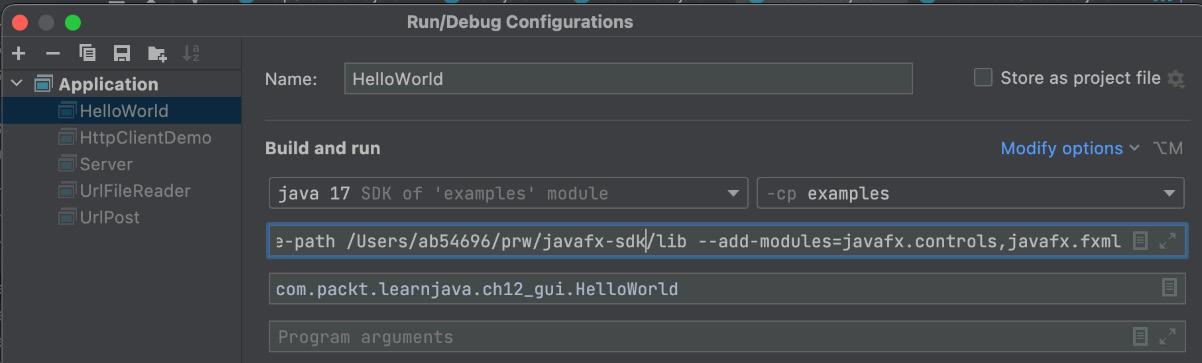``````mvn clean package
java --module-path /path/javafx-sdk/lib                 \
-cp target/examples-1.0-SNAPSHOT.jar:target/libs/* \
com.packt.learnjava.ch12_gui.HelloWorld
java --module-path /path/javafx-sdk/lib                  \
-cp target/examples-1.0-SNAPSHOT.jar:target/libs/*  \
com.packt.learnjava.ch12_gui.BlendEffect
java --module-path /path/javafx-sdk/lib                  \
-cp target/examples-1.0-SNAPSHOT.jar:target/libs/*  \
com.packt.learnjava.ch12_gui.OtherEffects``````

``````mvn clean package
java --module-path C:\path\javafx-sdk\lib                \
-cp target\examples-1.0-SNAPSHOT.jar;target\libs\*  \
com.packt.learnjava.ch12_gui.HelloWorld
java --module-path C:\path\javafx-sdk\lib                 \
-cp target\examples-1.0-SNAPSHOT.jar;target\libs\*   \
com.packt.learnjava.ch12_gui.BlendEffect
java --module-path C:\path\javafx-sdk\lib                  \
-cp target\examples-1.0-SNAPSHOT.jar;target\libs\*    \
com.packt.learnjava.ch12_gui.OtherEffects``````

JavaFX 的高级介绍到此结束。有了这个，我们进入最令人兴奋的（对于任何程序员）部分：编写代码。

# HelloWorld with JavaFX

``import javafx.application.Application;``
``import javafx.application.Platform;``
``import javafx.scene.control.Button;``
``import javafx.scene.layout.Pane;``
``import javafx.scene.text.Text;``
``import javafx.scene.Scene;``
``import javafx.stage.Stage;``
``public class HelloWorld extends Application {``
``  public static void main(String... args) {``
``      launch(args);``
``  }``
``  @Override``
``  public void start(Stage primaryStage) {``
``    Text txt = new Text("Hello, world!");``
``    txt.relocate(135, 40);``
``    Button btn = new Button("Exit");``
``    btn.relocate(155, 80);``
``    btn.setOnAction(e:> {``
``        System.out.println("Bye! See you later!");``
``        Platform.exit();``
``    });``
``    Pane pane = new Pane();``
``    pane.getChildren().addAll(txt, btn);``
``    primaryStage``
``        .setTitle("The primary stage (top-level container)");``
``    primaryStage.onCloseRequestProperty()``
``        .setValue(e:> System.out.println(``
``                                       "Bye! See you later!"));``
``    primaryStage.setScene(new Scene(pane, 350, 150));``
``    primaryStage.show();``
``  }``
``}``

`Stage` 对象的标题为`初级阶段（顶级容器）`。在单击窗口右上角的关闭窗口符号（x 按钮）时，还会为其分配一个操作：Linux 系统的左上角和 Windows 的右上角系统。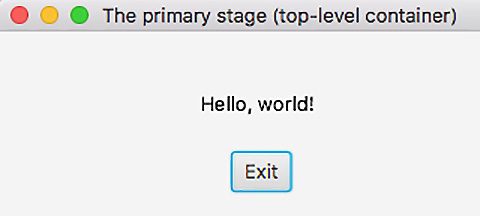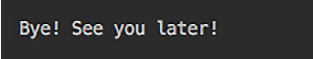``````@Override
public void stop(){
System.out.println(
"Doing what has to be done before closing");}``````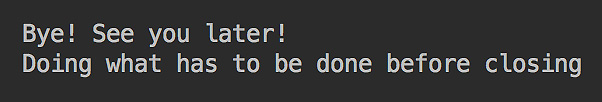# Control elements

``Text txt = new Text("Fill the form and click Submit");``
``TextField tfFirstName = new TextField();``
``TextField tfLastName = new TextField();``
``TextField tfAge = new TextField();``
``Button btn = new Button("Submit");``
``btn.setOnAction(e:> action(tfFirstName, tfLastName, tfAge));``

``void action(TextField tfFirstName, ``
``                TextField tfLastName, TextField tfAge ) {``
``    String fn = tfFirstName.getText();``
``    String ln = tfLastName.getText();``
``    String age = tfAge.getText();``
``    int a = 42;``
``    try {``
``        a = Integer.parseInt(age);``
``    } catch (Exception ex){}``
``    fn = fn.isBlank() ? "Nick" : fn;``
``    ln = ln.isBlank() ? "Samoylov" : ln;``
``    System.out.println("Hello, "+fn+" "+ln + ", age " + ``
``                                                      a + "!");``
``    Platform.exit();``
``}``

``GridPane grid = new GridPane();``
``grid.setAlignment(Pos.CENTER);``
``grid.setHgap(15);``
``grid.setVgap(5);``
``grid.setPadding(new Insets(20, 20, 20, 20));``

`GridPane` 布局窗格的行和列构成了可以在其中设置节点的单元格。节点可以跨越列和行。 `setAlignment()` 方法将网格的位置设置为场景的中心（默认位置是场景的左上角）。 `setHgap()``setVgap()` 方法设置列（水平）和行（垂直）之间的间距（以像素为单位） ）。 `setPadding()` 方法沿网格窗格的边界添加了一些空间。 `Insets()` 对象按顶部、右侧、底部和左侧的顺序设置值（以像素为单位）。

``int i = 0;``
``grid.add(txt,    1, i++, 2, 1);``
``GridPane.setHalignment(txt, HPos.CENTER);``
``grid.addRow(i++, new Label("First Name"), tfFirstName);``
``grid.addRow(i++, new Label("Last Name"),  tfLastName);``
``grid.addRow(i++, new Label("Age"), tfAge);``
``grid.add(btn,    1, i);``
``GridPane.setHalignment(btn, HPos.CENTER);``

`add()` 方法接受 三个或五个参数：

• 节点、列索引和行索引
• 节点、列索引、行索引、要跨越多少列、要跨越多少行

`setHalignment()` 方法设置节点在单元格中的位置。 `HPos` 枚举具有值 `LEFT``RIGHT``CENTER``addRow(int i, Node... nodes)` 方法接受行索引和节点的可变参数。我们用它来放置 `Label``TextField` 对象。

`start()` 方法的其余部分与 `HelloWorld` 示例非常相似（仅更改了标题和大小）：

``primaryStage.setTitle("Simple form example");``
``primaryStage.onCloseRequestProperty()``
``     .setValue(e -> System.out.println("Bye! See you later!"));``
``primaryStage.setScene(new Scene(grid, 300, 200));``
``primaryStage.show();``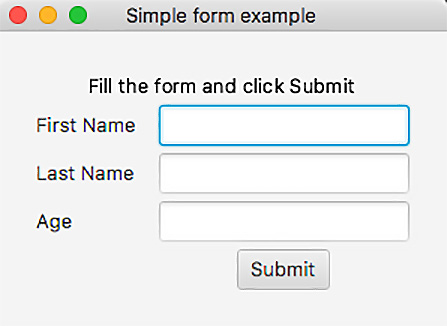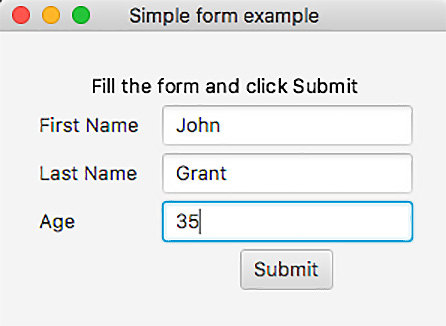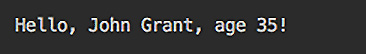``grid.setGridLinesVisible(true);``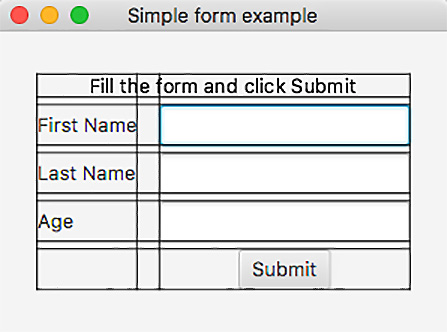`javafx.scene.layout` 包包含 `Pane` 等 24 个布局类（我们在 `HelloWorld` 示例)，`StackPane`（允许我们覆盖节点），`FlowPane`（允许节点的位置随着窗口大小的变化而流动）和 `AnchorPane` （保留 节点相对于其锚点的位置），仅举几例。 `VBox` 布局将在下一节中演示，Charts

# Charts

JavaFX 在 `javafx.scene.chart` 包中提供了 以下图表组件用于数据可视化：

• `LineChart`：在系列中的数据点之间添加 一条线。通常用于呈现一段时间内的趋势。
• `AreaChart`：类似于`LineChart`，但填充连接数据点和轴的线之间的区域。通常用于比较一段时间内的累计总数。
• `BarChart`：将数据呈现为矩形条。用于离散数据的可视化。
• `PieChart`：呈现一个分成多个段（用不同颜色填充）的圆，每个段代表一个值占总数的比例。我们将在本节中演示它。
• `BubbleChart`：将数据呈现为称为气泡的二维椭圆形，允许呈现三个参数。
• `ScatterChart`：按原样呈现一系列数据点。有助于识别是否存在聚类（数据相关性）。

``Text txt = new Text("Test results:");``
``PieChart pc = new PieChart();``
``pc.getData().add(new PieChart.Data("Succeed", 143));``
``pc.getData().add(new PieChart.Data("Failed" ,  12));``
``pc.getData().add(new PieChart.Data("Ignored",  18));``
``VBox vb = new VBox(txt, pc);``
``vb.setAlignment(Pos.CENTER);``
``vb.setPadding(new Insets(10, 10, 10, 10));``
``primaryStage.setTitle("A chart example");``
``primaryStage.onCloseRequestProperty()``
``      .setValue(e:> System.out.println("Bye! See you later!"));``
``primaryStage.setScene(new Scene(vb, 300, 300));``
``primaryStage.show();``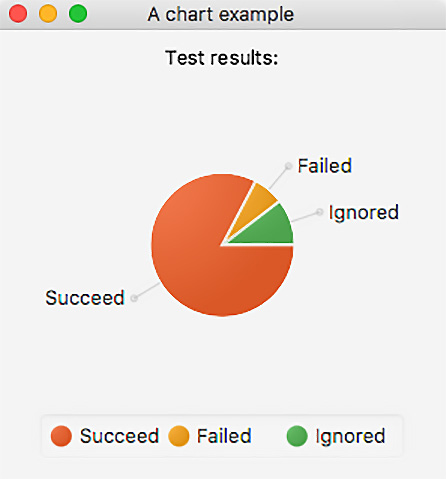`PieChart` 类以及任何其他图表具有其他几种方法，可用于呈现 更复杂和动态的数据以用户友好的 方式。

# Applying CSS

``scene.getStylesheets().add("/mystyle.css");``

`mystyle.css` 文件必须放在 `src/main/resources` 文件夹中。让我们这样做，将具有以下内容的 `mystyle.css` 文件添加到 `HelloWorld` 示例中：

``#text-hello {``
``  :fx-font-size: 20px;``
``   -fx-font-family: "Arial";``
``   -fx-fill: red;``
``}``
``.button {``
``   -fx-text-fill: white;``
``   -fx-background-color: slateblue;``
``}``

``Text txt = new Text("Hello, world!");``
``txt.setId("text-hello");``
``txt.relocate(115, 40);``
``Button btn = new Button("Exit");``
``btn.relocate(155, 80);``
``btn.setOnAction(e -> {``
``    System.out.println("Bye! See you later!");``
``    Platform.exit();``
``});``
``Pane pane = new Pane();``
``pane.getChildren().addAll(txt, btn);``
``Scene scene = new Scene(pane, 350, 150);``
``scene.getStylesheets().add("/mystyle.css");``
``primaryStage.setTitle("The primary stage (top-level container)");``
``primaryStage.onCloseRequestProperty()``
``   .setValue(e -> System.out.println("\nBye! See you later!"));``
``primaryStage.setScene(scene);``
``primaryStage.show();``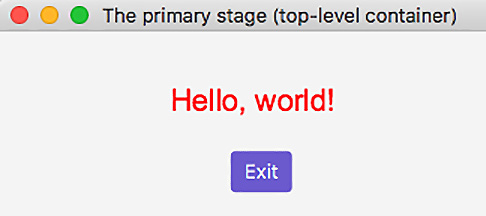``btn.setStyle("-fx-text-fill: white; -fx-background-color: red;");``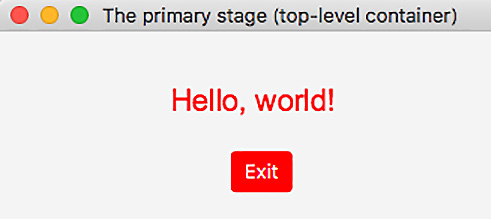# Using FXML

FXML 是一种 基于 XML 的语言，它允许构建用户界面并独立于应用程序（业务）逻辑（如就外观而言，或其他与演示相关的更改）。使用 FXML，您甚至无需编写一行 Java 代码即可设计用户界面。

FXML 没有模式，但它的功能反映了用于构建场景的 JavaFX 对象的 API。这意味着您可以使用 API 文档来了解 FXML 结构中允许使用哪些标签和属性。大多数时候，JavaFX 类可以用作标记，它们的属性可以用作属性。

``<?xml version="1.0" encoding="UTF-8"?>``
``<?import javafx.scene.Scene?>``
``<?import javafx.geometry.Insets?>``
``<?import javafx.scene.text.Text?>``
``<?import javafx.scene.control.Label?>``
``<?import javafx.scene.control.Button?>``
``<?import javafx.scene.layout.GridPane?>``
``<?import javafx.scene.control.TextField?>``
``<Scene fx:controller="com.packt.learnjava.ch12_gui.HelloWorldController"``
``       xmlns:fx="http://javafx.com/fxml"``
``       width="350" height="200">``
``    <GridPane alignment="center" hgap="15" vgap="5">``
``        <padding>``
``            <Insets top="20" right="20" bottom="20" left="20"/>``
``        </padding>``
``        <Text id="textFill" text="Fill the form and click ``
``         Submit" GridPane.rowIndex="0" GridPane.columnSpan="2">``
``            <GridPane.halignment>center</GridPane.halignment>``
``        </Text>``
``        <Label text="First name"``
``               GridPane.columnIndex="0" GridPane.rowIndex="1"/>``
``        <TextField fx:id="tfFirstName"``
``               GridPane.columnIndex="1" GridPane.rowIndex="1"/>``
``        <Label text="Last name"``
``               GridPane.columnIndex="0" GridPane.rowIndex="2"/>``
``        <TextField fx:id="tfLastName"``
``               GridPane.columnIndex="1" GridPane.rowIndex="2"/>``
``        <Label text="Age"``
``               GridPane.columnIndex="0" GridPane.rowIndex="3"/>``
``        <TextField fx:id="tfAge"``
``               GridPane.columnIndex="1" GridPane.rowIndex="3"/>``
``        <Button text="Submit"``
``                GridPane.columnIndex="1" GridPane.rowIndex="4"``
``                onAction="#submitClicked">``
``            <GridPane.halignment>center</GridPane.halignment>``
``        </Button>``
``    </GridPane>``
``</Scene>``

``try {``
``  ClassLoader classLoader =``
``             Thread.currentThread().getContextClassLoader();``
``  String file =``
``        classLoader.getResource("helloWorld.fxml").getFile();``
``  FXMLLoader lder = new FXMLLoader();``
``  lder.setLocation(new URL("file:" + file));``
``  Scene scene = lder.load();``
``  primaryStage.setTitle("Simple form example");``
``  primaryStage.setScene(scene);``
``  primaryStage.onCloseRequestProperty().setValue(e ->``
``                  System.out.println("\nBye! See you later!"));``
``  primaryStage.show();``
``} catch (Exception ex){``
``    ex.printStackTrace();``
``}``

`start()` 方法 只是加载 `helloWorld.fxml` 文件并设置阶段，后者与我们之前的示例完全相同。

``public class HelloWorldController {``
``    @FXML``
``    protected void submitClicked(ActionEvent e) {``
``    }``
``}``

``@FXML``
``private TextField tfFirstName;``
``@FXML``
``private TextField tfLastName;``
``@FXML``
``private TextField tfAge;``
``@FXML``
``protected void submitClicked(ActionEvent e) {``
``    String fn = tfFirstName.getText();``
``    String ln = tfLastName.getText();``
``    String age = tfAge.getText();``
``    int a = 42;``
``    try {``
``        a = Integer.parseInt(age);``
``    } catch (Exception ex) {``
``    }``
``    fn = fn.isBlank() ? "Nick" : fn;``
``    ln = ln.isBlank() ? "Samoylov" : ln;``
``    String hello = "Hello, " + fn + " " + ln + ", age " + ``
``                                                       a + "!";``
``    System.out.println(hello);``
``    Platform.exit();``
``}``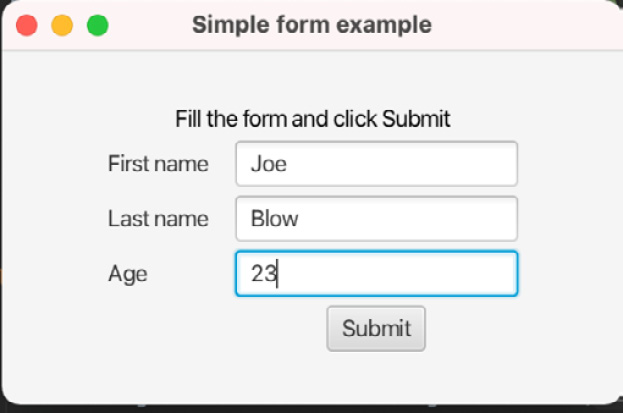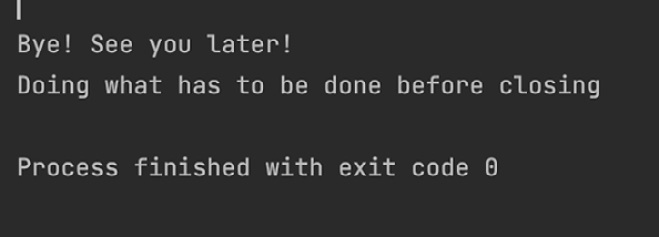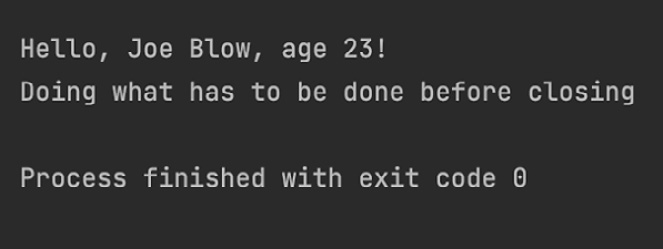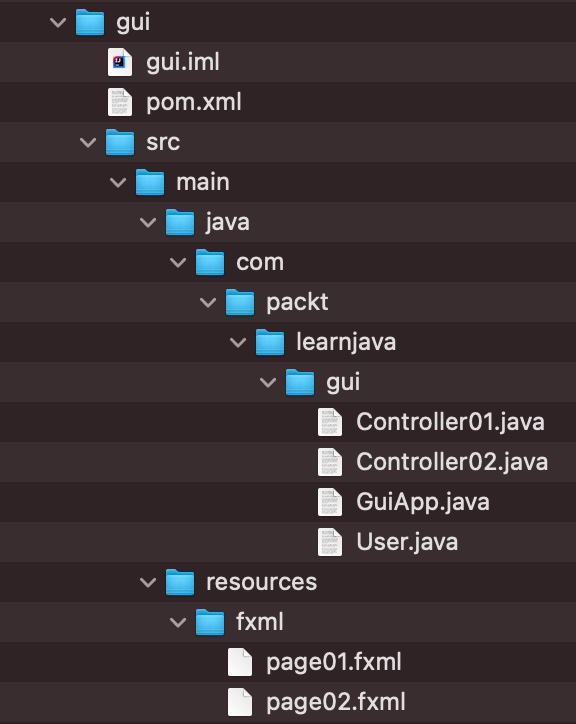``public class GuiApp extends Application {``
``    public static void main(String... args) {``
``        launch(args);``
``    }``
``    @Override``
``    public void stop(){``
``        System.out.println("Doing what has to be done...");``
``    }``
``    public void start(Stage primaryStage) {``
``        Controller01.start(primaryStage);``
``    }``
``}````    @FXML``
``    protected void submitClicked(ActionEvent e) {``
``        String fn = tfFirstName.getText();``
``        String ln = tfLastName.getText();``
``        String age = tfAge.getText();``
``        int a = 42;``
``        try {``
``            a = Integer.parseInt(age);``
``        } catch (Exception ex) {``
``        }``
``        fn = fn.isBlank() ? "Nick" : fn;``
``        ln = ln.isBlank() ? "Samoylov" : ln;``
``        Controller02.goToPage2(new User(a, fn, ln));``
`` ``
``        Node source = (Node) e.getSource();``
``        Stage stage = (Stage) source.getScene().getWindow();``
``        stage.close();``
``    }``

`Controller02.goToPage2()` 方法 如下所示：

``public static void goToPage2(User user) {``
``  try {``
``    ClassLoader classLoader =``
``             Thread.currentThread().getContextClassLoader();``
``    String file = classLoader.getResource("fxml" + ``
``                  File.separator + "page02.fxml").getFile();``
``    FXMLLoader loader = new FXMLLoader();``
``    loader.setLocation(new URL("file:" + file));``
``    Scene scene = loader.load();``
`` ``
``    Controller02 c = loader.getController();``
``    String hello = "Hello, " + user.getFirstName() + " " + ``
``        user.getLastName() + ", age " + user.getAge() + "!";``
``    c.textHello.setText(hello);``
`` ``
``    Stage primaryStage = new Stage();``
``    primaryStage.setTitle("Second page of GUI App");``
``    primaryStage.setScene(scene);``
``    primaryStage.onCloseRequestProperty()``
``        .setValue(e -> {``
``                          System.out.println("\nBye!");``
``                          Platform.exit();``
``                       });``
``    primaryStage.show();``
``  } catch (Exception ex) {``
``       ex.printStackTrace();``
``  }``
``}``

``<?xml version="1.0" encoding="UTF-8"?>``
``<?import javafx.scene.Scene?>``
``<?import javafx.geometry.Insets?>``
``<?import javafx.scene.text.Text?>``
``<?import javafx.scene.layout.GridPane?>``
``<Scene fx:controller="com.packt.lernjava.gui.Controller02"``
``       xmlns:fx="http://javafx.com/fxml"``
``       width="350" height="150">``
``    <GridPane alignment="center" hgap="15" vgap="5">``
``        <padding>``
``            <Insets top="20" right="20" bottom="20" left="20"/>``
``        </padding>``
``        <Text fx:id="textHello"``
``              GridPane.rowIndex="0" GridPane.columnSpan="2">``
``            <GridPane.halignment>center</GridPane.halignment>``
``        </Text>``
``        <Text id="textDo" text="Do what has to be done here"``
``              GridPane.rowIndex="1" GridPane.columnSpan="2">``
``            <GridPane.halignment>center</GridPane.halignment>``
``        </Text>``
``    </GridPane>``
``</Scene>``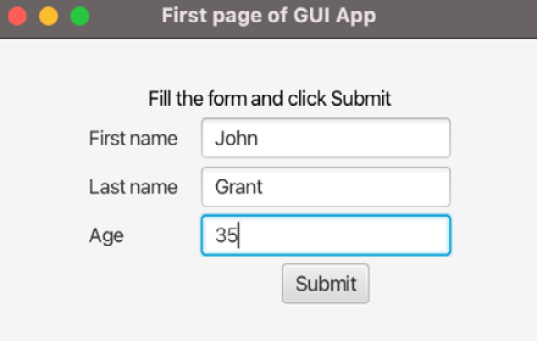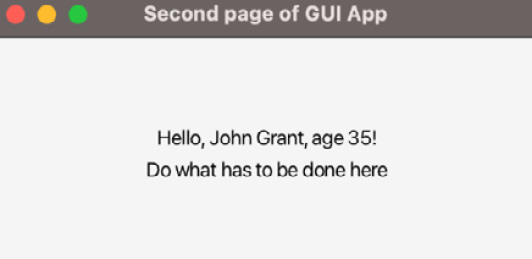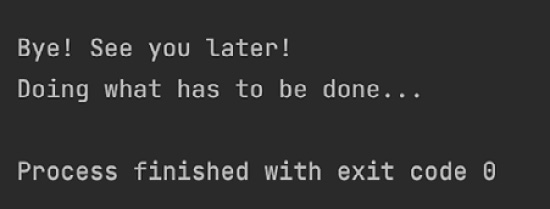`stop()` 方法按预期工作。

# Embedding HTML

1. 将以下依赖项添加到 `pom.xml` 文件中：
``<依赖>    <groupId>org.openjfx</groupId>    <artifactId>javafx-web</artifactId>    <版本>11.0.2</版本> </dependency>``

`javafx-web` 的版本通常与 Java 版本保持同步，但在撰写本文时，`javafx-web` 尚未发布，因此我们正在使用最新的可用版本，11.0.2

1. 由于 `javafx-web` 使用 `com.sun.*` 包，它已从 Java 9 中删除（https://docs.oracle.com/javase/9​​/migrate/toc.htm#JSMIG-GUID-F7696E02-A1FB-4D5A-B1F2-89E7007D4096)，访问 `com.sun.*` 来自 Java 9+ 的包，除了 `--module-path``--add-modules`，在 `HtmlWebView` 类的 `Run/Debug Configuration` 中的 JavaFX 基础部分中进行了描述` ch12_gui` 包（对于 Windows，将斜杠符号更改为反斜杠）：
``--add-exports javafx.graphics/com.sun.javafx.sg.prism=ALL-UNNAMED  --add-exports javafx.graphics/com.sun.javafx.scene=ALL-UNNAMED  --add-exports javafx.graphics/com.sun.javafx.util=ALL-UNNAMED  --add-exports javafx.base/com.sun.javafx.logging=ALL-UNNAMED  --add-exports javafx.graphics/com.sun.prism=ALL-UNNAMED  --add-exports javafx.graphics/com.sun.glass.ui=ALL-UNNAMED  --add-exports javafx.graphics/com.sun.javafx.geom.transform=ALL-UNNAMED  --add-exports javafx.graphics/com.sun.javafx.tk=ALL-UNNAMED  --add-exports javafx.graphics/com.sun.glass.utils=ALL-UNNAMED  --add-exports javafx.graphics/com.sun.javafx.font=ALL-UNNAMED  --add-exports javafx.graphics/com.sun.javafx.application=ALL-UNNAMED  --add-exports javafx.controls/com.sun.javafx.scene.control=ALL-UNNAMED  --add-exports javafx.graphics/com.sun.javafx.scene.input=ALL-UNNAMED  --add-exports javafx.graphics/com.sun.javafx.geom=ALL-UNNAMED  --add-exports javafx.graphics/com.sun.prism.paint=ALL-UNNAMED  --add-exports javafx.graphics/com.sun.scenario.effect=ALL-UNNAMED  --add-exports javafx.graphics/com.sun.javafx.text=ALL-UNNAMED  --add-exports javafx.graphics/com.sun.javafx.iio=ALL-UNNAMED --add-exports javafx.graphics/com.sun.scenario.effect.impl.prism=ALL-UNNAMED --add-exports javafx.graphics/com.sun.javafx.scene.text=ALL-UNNAMED``
2. 要从命令行执行 `HtmlWebView` 类，请转到 `examples` 文件夹并在 Unix/Linux/macOS 上使用以下命令系统（不要忘记将 `/path/JavaFX` 替换为 包含 JavaFX SDK 的文件夹的实际路径）：
``mvn clean package java --module-path /path/javaFX/lib --add-modules=javafx.controls,javafx.fxml --add-exports javafx.graphics/com.sun.javafx.sg。 prism=ALL-UNNAMED --add-exports javafx.graphics/com.sun.javafx.scene=ALL-UNNAMED --add-exports javafx.graphics/com.sun.javafx.util=ALL-UNNAMED --add-exports javafx.base/com.sun.javafx.logging=ALL-UNNAMED --add-exports javafx.graphics/com.sun.prism=ALL-UNNAMED --add-exports javafx.graphics/com.sun.glass.ui= ALL-UNNAMED --add-exports javafx.graphics/com.sun.javafx.geom.transform=ALL-UNNAMED --add-exports javafx.graphics/com.sun.javafx.tk=ALL-UNNAMED --add-exports javafx.graphics/com.sun.glass.utils=ALL-UNNAMED  --add-exports javafx.graphics/com.sun.javafx.font=ALL-UNNAMED  --add-exports javafx.graphics/ com.sun.javafx.application=ALL-UNNAMED --add-exports javafx.controls/com.sun.javafx.scene.control=ALL-UNNAMED --add-exports javafx.graphics/com.sun.javafx.scene。输入=全部未命名 --add-exports javafx.graphics/com.sun.javafx.ge om=ALL-UNNAMED  --add-exports javafx.graphics/com.sun.prism.paint=ALL-UNNAMED  --add-exports javafx.graphics/com.sun.scenario.effect=ALL-未命名 --add-exports javafx.graphics/com.sun.javafx.text=ALL-UNNAMED --add-exports javafx.graphics/com.sun.javafx.iio=ALL-UNNAMED --add-exports javafx.graphics/ com.sun.scenario.effect.impl.prism=ALL-UNNAMED --add-exports javafx.graphics/com.sun.javafx.scene.text=ALL-UNNAMED  ; -cp target/examples-1.0-SNAPSHOT.jar com.packt.learnjava.ch12_gui.HtmlWebView``
3. 在 Windows 上，相同的 命令如下所示（不要忘记将 `C:\path\JavaFX` 替换为实际路径到包含 JavaFX SDK 的文件夹）：
``mvn clean package java --module-path C:\path\JavaFX\lib --add-modules=javafx.controls,javafx.fxml --add-exports javafx.graphics\com.sun.javafx。 sg.prism=ALL-UNNAMED --add-exports javafx.graphics\com.sun.javafx.scene=ALL-UNNAMED --add-exports javafx.graphics\com.sun.javafx.util=ALL-UNNAMED --add -exports javafx.base\com.sun.javafx=ALL-UNNAMED --add-exports javafx.base\com.sun.javafx.logging=ALL-UNNAMED --add-exports javafx.graphics\com.sun.prism= ALL-UNNAMED --add-exports javafx.graphics\com.sun.glass.ui=ALL-UNNAMED --add-exports javafx.graphics\com.sun.javafx.geom.transform=ALL-UNNAMED --add-exports javafx.graphics\com.sun.javafx.tk=ALL-UNNAMED --add-exports javafx.graphics\com.sun.glass.utils=ALL-UNNAMED  --add-exports javafx.graphics\com.sun .javafx.font=ALL-UNNAMED  --add-exports javafx.graphics\com.sun.javafx.application=ALL-UNNAMED --add-exports javafx.controls\com.sun.javafx.scene.control= ALL-UNNAMED --add-exports javafx.graphics\com.sun.javafx.scene.input=ALL-未命名 --add-exports javafx.graphics\com.sun.javafx.geom=ALL-UNNAMED  --add-exports javafx.graphics\com.sun.prism.paint=ALL-UNNAMED  --add -exports javafx.graphics\com.sun.scenario.effect=ALL-UNNAMED --add-exports javafx.graphics\com.sun.javafx.text=ALL-UNNAMED --add-exports javafx.graphics\com.sun。 javafx.iio=ALL-UNNAMED --add-exports javafx.graphics\com.sun.scenario.effect.impl.prism=ALL-UNNAMED --add-exports javafx.graphics\com.sun.javafx.scene.text= ALL-UNNAMED  -cp target\examples-1.0-SNAPSHOT.jar com.packt.learnjava.ch12_gui.HtmlWebView``

`HtmlWebView` 类也包含几个 `start()` 方法。如JavaFX基础部分所述，一一重命名并执行它们

``WebView wv = new WebView();``
``WebEngine we = wv.getEngine();``
``String html = ``
``        "<html><center><h2>Hello, world!</h2></center></html>";``
``we.loadContent(html, "text/html");``
``Scene scene = new Scene(wv, 200, 60);``
``primaryStage.setTitle("My HTML page");``
``primaryStage.setScene(scene);``
``primaryStage.onCloseRequestProperty()``
``     .setValue(e -> System.out.println("Bye! See you later!"));``
``primaryStage.show();````Text txt = new Text("Below is the embedded HTML:");``
``WebView wv = new WebView();``
``WebEngine we = wv.getEngine();``
``String html = ``
``      "<html><center><h2>Hello, world!</h2></center></html>";``
``we.loadContent(html, "text/html");``
``VBox vb = new VBox(txt, wv);``
``vb.setSpacing(10);``
``vb.setAlignment(Pos.CENTER);``
``vb.setPadding(new Insets(10, 10, 10, 10));``
``Scene scene = new Scene(vb, 300, 120);``
``primaryStage.setScene(scene);``
``primaryStage.setTitle("JavaFX with embedded HTML");``
``primaryStage.onCloseRequestProperty()``
``     .setValue(e -> System.out.println("Bye! See you later!"));``
``primaryStage.show();``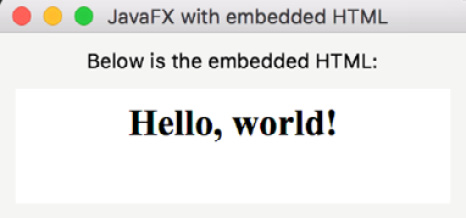``<!DOCTYPE html>``
``<html lang="en">``
``<head>``
``    <meta charset="UTF-8">``
``    <title>The Form</title>``
``</head>``
``<body>``
``<form action="http://someServer:port/formHandler" method="post">``
``  <table>``
``    <tr>``
``      <td><label for="firstName">First name:</label></td>``
``      <td><input type="text" id="firstName" name="firstName">``
``      </td>``
``    </tr>``
``    <tr>``
``      <td><label for="lastName">Last name:</label></td>``
``      <td><input type="text" id="lastName" name="lastName">``
``      </td>``
``    </tr>``
``    <tr>``
``      <td><label for="age">Age:</label></td>``
``      <td><input type="text" id="age" name="age"></td>``
``    </tr>``
``    <tr>``
``      <td></td>``
``      <td align="center">``
``          <button id="submit" name="submit">Submit</button>``
``      </td>``
``    </tr>``
``  </table>``
``</form>``
``</body>``
``</html>``

``ClassLoader classLoader =``
``              Thread.currentThread().getContextClassLoader();``
``String file = classLoader.getResource("form.html").getFile();``
``Text txt = new Text("Fill the form and click Submit");``
``WebView wv = new WebView();``
``WebEngine we = wv.getEngine();``
``File f = new File(file);``
``we.load(f.toURI().toString());``
``VBox vb = new VBox(txt, wv);``
``vb.setSpacing(10);``
``vb.setAlignment(Pos.CENTER);``
``vb.setPadding(new Insets(10, 10, 10, 10));``
``Scene scene = new Scene(vb, 300, 200);``
``primaryStage.setScene(scene);``
``primaryStage.setTitle("JavaFX with embedded HTML");``
``primaryStage.onCloseRequestProperty()``
``     .setValue(e -> System.out.println("Bye! See you later!"));``
``primaryStage.show();``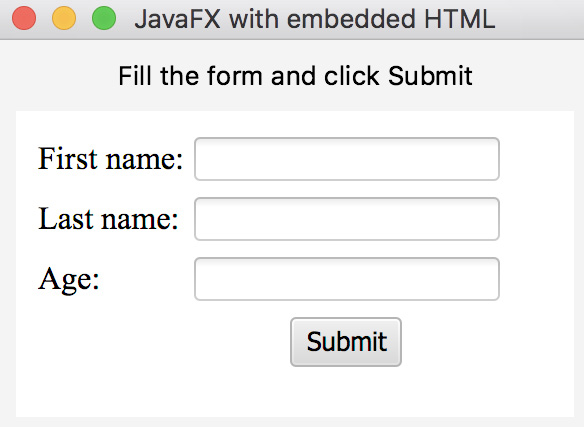``Text txt = new Text("Enjoy searching the Web!");``
``WebView wv = new WebView();``
``WebEngine we = wv.getEngine();``
``we.load("http://www.google.com");``
``VBox vb = new VBox(txt, wv);``
``vb.setSpacing(20);``
``vb.setAlignment(Pos.CENTER);``
``vb.setStyle("-fx-font-size: 20px;-fx-background-color: lightblue;");``
``vb.setPadding(new Insets(10, 10, 10, 10));``
``Scene scene = new Scene(vb,750,500);``
``primaryStage.setScene(scene);``
``primaryStage.setTitle(``
``                   "JavaFX with the window to another server");``
``primaryStage.onCloseRequestProperty()``
``     .setValue(e -> System.out.println("Bye! See you later!"));``
``primaryStage.show();``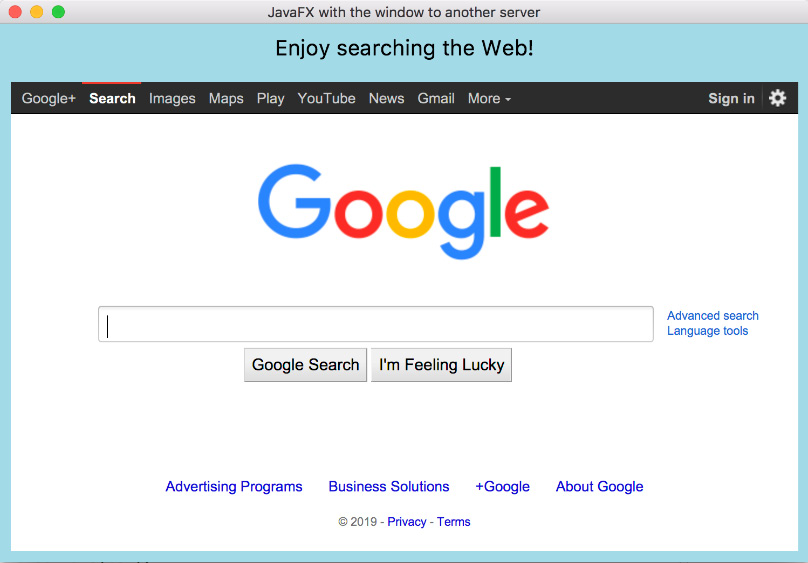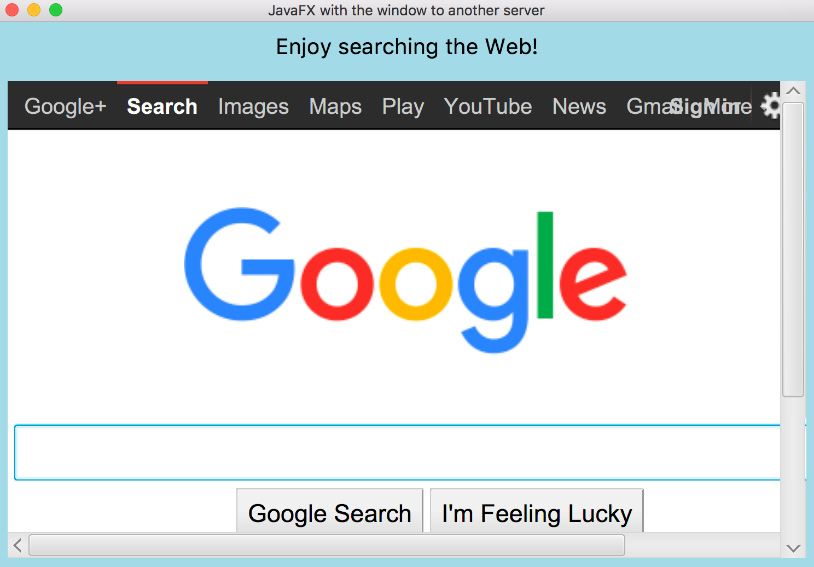``wv.setFontScale(1.5);``
``we.setUserStyleSheetLocation("mystyle.css");``

``Document document = we.getDocument();``

``WebHistory history = we.getHistory();  ``
``int currInd = history.getCurrentIndex(); ``
``history.go(-1);``
``history.go( 1);``

``WebHistory history = we.getHistory();``
``ObservableList<WebHistory.Entry> entries = ``
``                                          history.getEntries();``
``for(WebHistory.Entry entry: entries){``
``    String url = entry.getUrl();``
``    String title = entry.getTitle();``
``    Date date = entry.getLastVisitedDate();``
``}``

# Playing media

``ClassLoader classLoader =``
``             Thread.currentThread().getContextClassLoader();``
``String file = classLoader.getResource("packt.png").getFile();``
``Text txt = new Text("What a beautiful image!");``
``FileInputStream input = new FileInputStream(file);``
``Image image = new Image(input);``
``ImageView iv = new ImageView(image);``
``VBox vb = new VBox(txt, iv);``
``vb.setSpacing(20);``
``vb.setAlignment(Pos.CENTER);``
``vb.setPadding(new Insets(10, 10, 10, 10));``
``Scene scene = new Scene(vb, 300, 200);``
``primaryStage.setScene(scene);``
``primaryStage.setTitle("JavaFX with embedded HTML");``
``primaryStage.onCloseRequestProperty()``
``     .setValue(e -> System.out.println("Bye! See you later!"));``
``primaryStage.show();``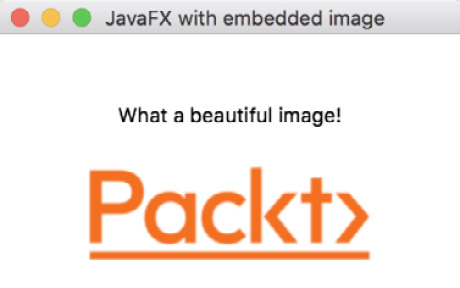• `AAC`高级音频编码音频压缩
• `H.264/AVC`: H.264/MPEG-4 Part 10 / AVC (高级视频编码) 视频压缩
• `MP3`：原始 MPEG-1、2 和 2.5 音频；第一层、第二层和第三层
• `PCM`：未压缩的原始音频样本

``javafx.scene.media.Media;``
``javafx.scene.media.MediaPlayer;``
``javafx.scene.media.MediaView;``

`Media` 类表示媒体的来源。 `MediaPlayer` 类提供了所有控制媒体播放的方法：`play()`, `stop ()``pause()``setVolume()` 等。您还可以指定媒体应播放的次数。 `MediaView` 类扩展了 `Node` 类并且可以添加到场景中。它提供媒体播放器正在播放的媒体的视图，并负责媒体外观。

``Text txt1 = new Text("What a beautiful music!");``
``Text txt2 = ``
``   new Text("If you don't hear music, turn up the volume.");``
``ClassLoader classLoader =``
``             Thread.currentThread().getContextClassLoader();``
``String file = classLoader.getResource("jb.mp3").getFile();``
``File f = new File(file);``
``Media m = new Media(f.toURI().toString());``
``MediaPlayer mp = new MediaPlayer(m);``
``MediaView mv = new MediaView(mp);``
``VBox vb = new VBox(txt1, txt2, mv);``
``vb.setSpacing(20);``
``vb.setAlignment(Pos.CENTER);``
``vb.setPadding(new Insets(10, 10, 10, 10));``
``Scene scene = new Scene(vb, 350, 100);``
``primaryStage.setScene(scene);``
``primaryStage.setTitle("JavaFX with embedded media player");``
``primaryStage.onCloseRequestProperty()``
``     .setValue(e -> System.out.println("Bye! See you later!"));``
``primaryStage.show();``
``mp.play();``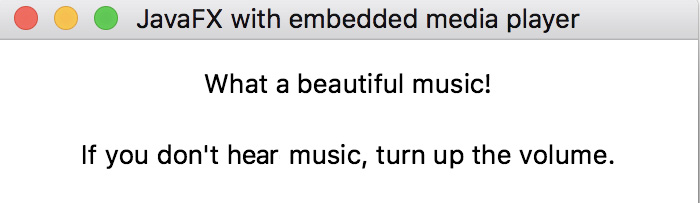`sea.mp4` 电影 文件 可以类似地播放（`HtmlWebView` 类的 class="literal">start6() 方法）：

``Text txt = new Text("What a beautiful movie!");``
``ClassLoader classLoader =``
``             Thread.currentThread().getContextClassLoader();``
``String file = classLoader.getResource("sea.mp4").getFile(); ``
``File f = new File(file);``
``Media m = new Media(f.toURI().toString());``
``MediaPlayer mp = new MediaPlayer(m);``
``MediaView mv = new MediaView(mp);``
``VBox vb = new VBox(txt, mv);``
``vb.setSpacing(20);``
``vb.setAlignment(Pos.CENTER);``
``vb.setPadding(new Insets(10, 10, 10, 10));``
``Scene scene = new Scene(vb, 650, 400);``
``primaryStage.setScene(scene);``
``primaryStage.setTitle("JavaFX with embedded media player");``
``primaryStage.onCloseRequestProperty()``
``     .setValue(e -> System.out.println("Bye! See you later!"));``
``primaryStage.show();``
``mp.play();``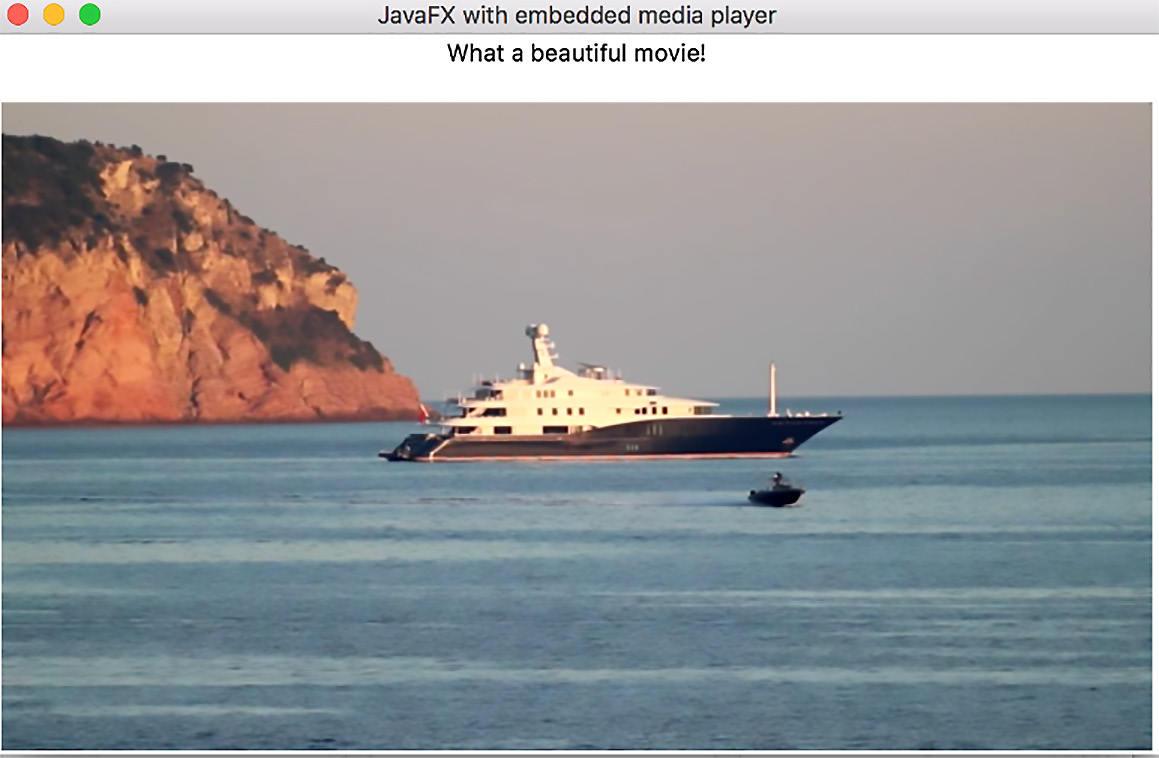``Text txt1 = new Text("What a beautiful movie and sound!");``
``Text txt2 = new Text("If you don't hear music, turn up the volume.");``
``ClassLoader classLoader =``
``             Thread.currentThread().getContextClassLoader();``
``String file = classLoader.getResource("jb.mp3").getFile(); ``
``File fs = new File(file);``
``Media ms = new Media(fs.toURI().toString());``
``MediaPlayer mps = new MediaPlayer(ms);``
``MediaView mvs = new MediaView(mps);``
``File fv = ``
``     new File(classLoader.getResource("sea.mp4").getFile());``
``Media mv = new Media(fv.toURI().toString());``
``MediaPlayer mpv = new MediaPlayer(mv);``
``MediaView mvv = new MediaView(mpv);``
``VBox vb = new VBox(txt1, txt2, mvs, mvv);``
``vb.setSpacing(20);``
``vb.setAlignment(Pos.CENTER);``
``vb.setPadding(new Insets(10, 10, 10, 10));``
``Scene scene = new Scene(vb, 650, 500);``
``primaryStage.setScene(scene);``
``primaryStage.setTitle("JavaFX with embedded media player");``
``primaryStage.onCloseRequestProperty()``
``     .setValue(e -> System.out.println("Bye! See you later!"));``
``primaryStage.show();``
``mpv.play();``
``mps.play();``

`javafx.scene.effects` 包包含 许多允许向节点添加各种效果的类：

• `Blend`：使用预定义的 `BlendModes` 之一组合来自两个来源（通常是图像）的像素
• `Bloom`：使输入图像更亮，使其看起来发光
• `BoxBlur`：为图像添加模糊
• `ColorAdjust`：允许调整图像的色调、饱和度、亮度和对比度
• `ColorInput`：渲染一个填充了给定颜料的矩形区域
• `DisplacementMap`：将每个像素移动指定距离
• `DropShadow`：在内容后面渲染给定内容的阴影
• `GaussianBlur`：使用特定（高斯）方法添加模糊
• `发光`：使输入图像看起来发光
• `InnerShadow`：在框架内创建阴影
• `Lighting`：模拟光源照射在内容上，使平面物体看起来更逼真
• `MotionBlur`：模拟运动中看到的给定内容
• `PerspectiveTransform`：转换透视图中的内容
• `Reflection`：在实际输入内容下方呈现输入的反射版本
• `SepiaTone`：产生棕褐色调效果，类似于古董照片的外观
• `Shadow`：创建带有模糊边缘的内容的单色副本

• opacity 属性的值：这定义了可以通过图像看到多少； opacity 值 0.0 表示图像完全透明，而 opacity 值 1.0 表示图像后面什么都看不到。
• 每种颜色的 alpha 值和强度：这将颜色的透明度定义为 0.0-1.0 或 0-255 范围内的双精度值。
• 混合模式，由 BlendMode 枚举值定义：根据每种颜色的模式、不透明度和 alpha 值，结果可能还取决于图像的顺序添加到场景中；第一个添加的图像称为底部输入，而重叠图像中的第二个称为顶部输入。如果顶部输入完全不透明，则底部输入被顶部输入隐藏。

• `ADD`：将顶部输入的颜色和 alpha 分量添加到底部输入的分量中。
• `BLUE`：将底部输入的蓝色分量替换为顶部输入的蓝色分量；其他颜色分量不受影响。
• `COLOR_BURN`：底部输入颜色分量的倒数除以顶部输入颜色分量，然后将所有这些分量反转以产生结果颜色。
• `COLOR_DODGE`：底部输入颜色分量除以顶部输入颜色分量的倒数以产生结果颜色。
• `DARKEN`：从两个输入中选择较暗的颜色分量来生成结果颜色。
• `DIFFERENCE`：从两个输入中较暗的颜色分量从较亮的分量中减去，以产生结果颜色。
• `EXCLUSION`：将两个输入的颜色分量相乘和加倍，然后从底部输入颜色分量的总和中减去，以产生结果颜色。
• `GREEN`：将底部输入的绿色分量替换为顶部输入的绿色分量；其他颜色分量不受影响。
• `HARD_LIGHT`：输入颜色分量是相乘还是筛选，取决于顶部输入颜色。
• `LIGHTEN`：选择来自两个输入的颜色分量中较亮的一个来产生结果颜色。
• `MULTIPLY`：第一个输入的 颜色分量乘以第二个输入的颜色分量。
• `OVERLAY`：根据底部输入颜色，输入颜色分量是相乘还是加网。
• `RED`：将底部输入的红色分量替换为顶部输入的红色分量；其他颜色分量不受影响。
• `SCREEN`：来自两个输入的颜色分量被反转，相互相乘，并且该结果再次反转以产生结果颜色。
• `SOFT_LIGHT`：输入颜色分量变暗或变亮，取决于顶部输入颜色。
• `SRC_ATOP`：位于底部输入内部的顶部输入部分与底部输入混合。
• `SRC_OVER`：顶部输入与底部输入混合。

``Circle createCircle(){``
``    Circle c = new Circle();``
``    c.setFill(Color.rgb(255, 0, 0, 0.5));``
``    c.setRadius(25);``
``    return c;``
``}``
``Rectangle createSquare(){``
``    Rectangle r = new Rectangle();``
``    r.setFill(Color.rgb(0, 0, 255, 1.0));``
``    r.setWidth(50);``
``    r.setHeight(50);``
``    return r;``
``}``

``Node c = createCircle();``
``Node s = createSquare();``
``Node g = new Group(s, c);``

``Node c = createCircle();``
``Node s = createSquare();``
``Node g = new Group(c, s);``

``Blend blnd = new Blend();``
``blnd.setMode(BlendMode.MULTIPLY);``
``Node c = createCircle();``
``Node s = createSquare();``
``Node g = new Group(s, c);``
``g.setEffect(blnd);``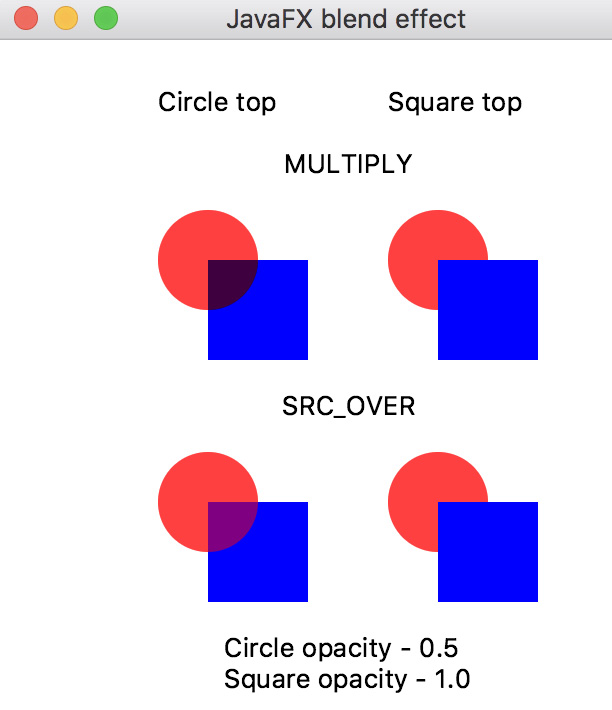``Node c = createCircle();``
``Node s = createSquare();``
``Node g = new Group(c, s);``
``g.setBlendMode(BlendMode.MULTIPLY);``

`start()` 方法中找到以下代码：

``      Node[] node = setEffectOnGroup(bm1, bm2);``
``      //Node[] node = setModeOnGroup(bm1, bm2);``

``      //Node[] node = setEffectOnGroup(bm1, bm2);``
``      Node[] node = setModeOnGroup(bm1, bm2);``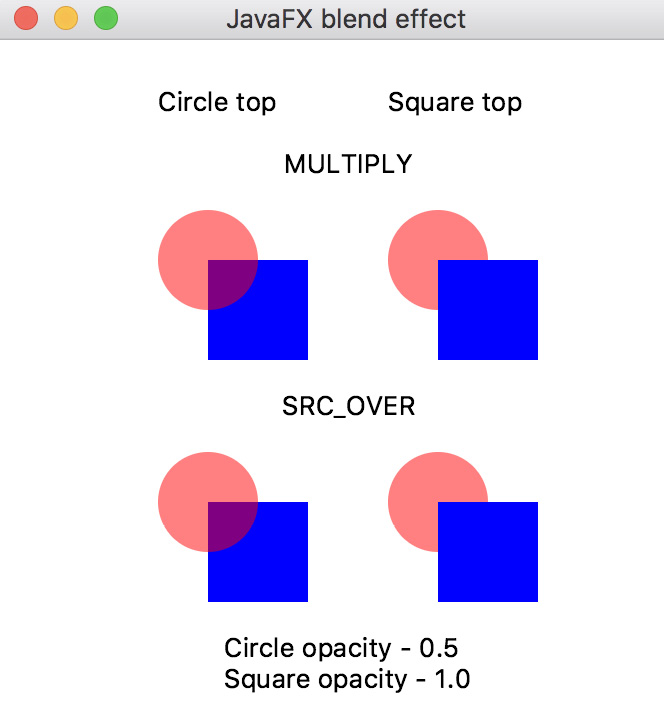``Blend blnd = new Blend();``
``blnd.setMode(BlendMode.MULTIPLY);``
``Node c = createCircle();``
``Node s = createSquare();``
``c.setEffect(blnd);``
``Node g = new Group(s, c);``

`start()` 方法中找到以下代码：

``      Node[] node = setModeOnGroup(bm1, bm2);``
``      //Node[] node = setEffectOnCircle(bm1, bm2);``

``      //Node[] node = setModeOnGroup(bm1, bm2);``
``      Node[] node = setEffectOnCircle(bm1, bm2);``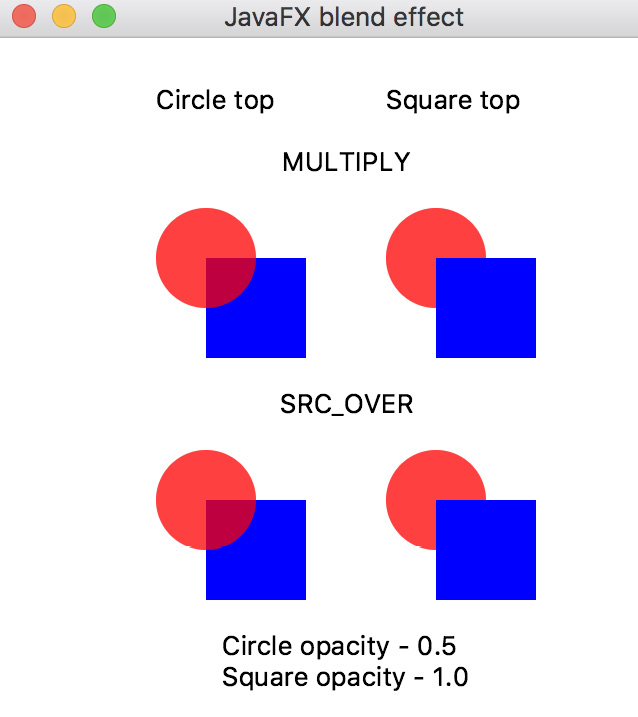``Blend blnd = new Blend();``
``blnd.setMode(BlendMode.MULTIPLY);``
``Node c = createCircle();``
``Node s = createSquare();``
``s.setEffect(blnd);``
``Node g = new Group(s, c);``

`start()` 方法中找到以下代码：

``      Node[] node = setEffectOnCircle(bm1, bm2);``
``      //Node[] node = setEffectOnSquare(bm1, bm2);``

``      //Node[] node = setEffectOnCircle(bm1, bm2);``
``      Node[] node = setEffectOnSquare(bm1, bm2); ``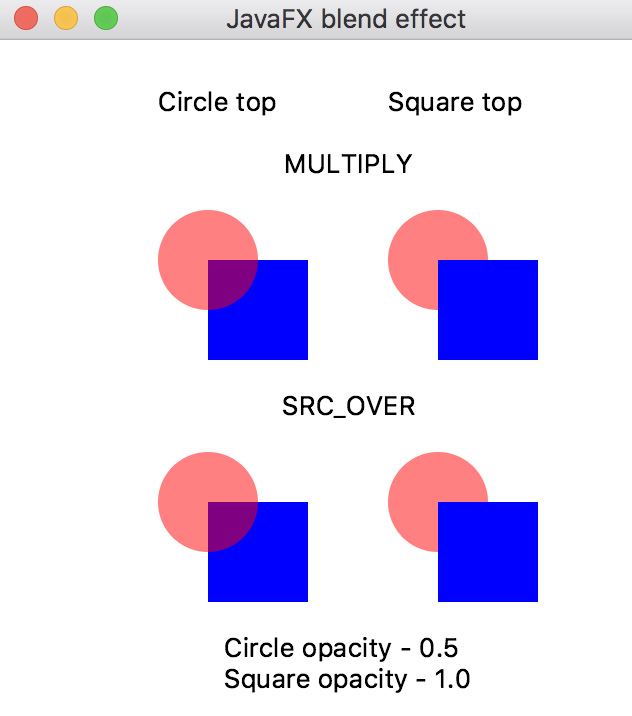`MULTIPLY``SRC_OVER` 模式之间没有 区别，但是红色颜色与我们在圆圈上设置效果时不同。

``Node c = createCircle();``
``Node s = createSquare();``
``c.setBlendMode(BlendMode.MULTIPLY);``

`start()` 方法中找到以下代码：

``      Node[] node = setEffectOnSquare(bm1, bm2);``
``      //Node[] node = setModeOnCircle(bm1, bm2);``

``      //Node[] node = setEffectOnSquare(bm1, bm2);``
``      Node[] node = setModeOnCircle(bm1, bm2);``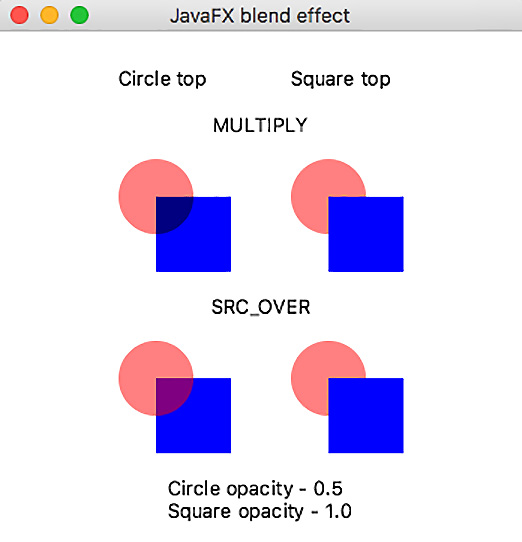`start()` 方法中找到以下 代码：

``      Node[] node = setModeOnCircle(bm1, bm2);``
``      //Node[] node = setModeOnSquare(bm1, bm2);``

``      //Node[] node = setModeOnCircle(bm1, bm2);``
``      Node[] node = setModeOnSquare(bm1, bm2);``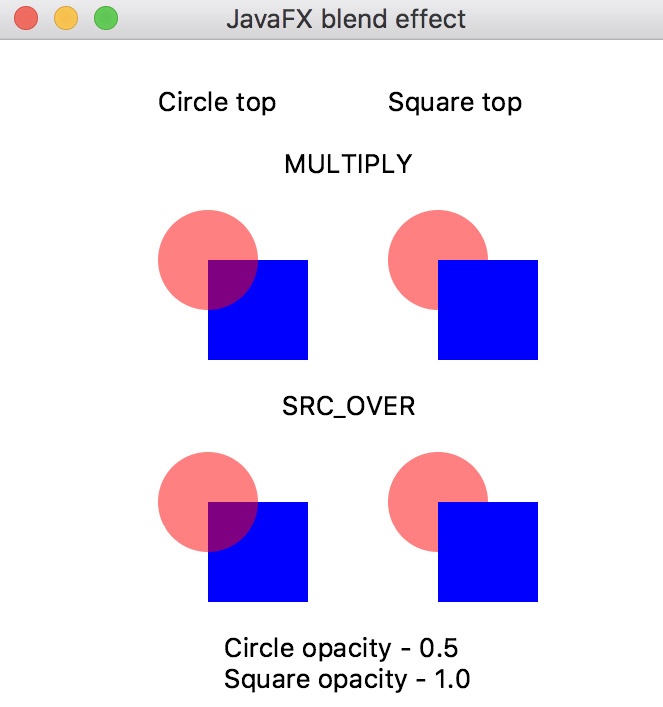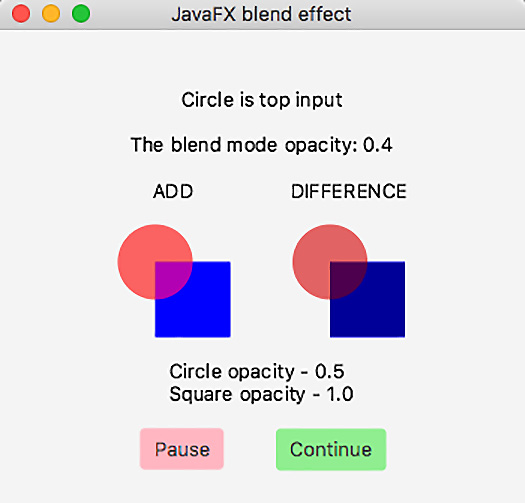``ClassLoader classLoader = ``
``              Thread.currentThread().getContextClassLoader(); ``
``String file = classLoader.getResource("packt.png").getFile(); FileInputStream inputP = new FileInputStream(file);``
``Image imageP = new Image(inputP);``
``ImageView ivP = new ImageView(imageP);``
``String file2 = classLoader.getResource("mount.jpeg").getFile(); FileInputStream inputM = new FileInputStream(file2);``
``Image imageM = new Image(inputM);``
``ImageView ivM = new ImageView(imageM);``
``ivM.setPreserveRatio(true);``
``ivM.setFitWidth(300);``

``Button btnP = new Button("Pause");``
``btnP.setOnAction(e1 -> et.pause());``
``btnP.setStyle("-fx-background-color: lightpink;");``
``Button btnC = new Button("Continue");``
``btnC.setOnAction(e2 -> et.cont());``
``btnC.setStyle("-fx-background-color: lightgreen;");``

`et` 对象是 `EffectsThread` 线程的对象：

``EffectsThread et = new EffectsThread(txt, ivM, ivP);``

``public void run(){``
``    try {``
``        for(String effect: effects){``
``            for(int i = 0; i < 11; i++){``
``                double d = Math.round(i * 0.1 * 10.0) / 10.0;``
``                Effect e = createEffect(effect, d, txt);``
``                ivM.setEffect(e);``
``                ivP.setEffect(e);``
``                TimeUnit.SECONDS.sleep(1);``
``                if(pause){``
``                    while(true){``
``                        TimeUnit.SECONDS.sleep(1);``
``                        if(!pause){``
``                            break;``
``                        }``
``                    }``
``                }``
``            }``
``        }``
``        Platform.exit();``
``    } catch (Exception ex){``
``        ex.printStackTrace();``
``    }``
``}``

``GridPane grid = new GridPane();``
``grid.setAlignment(Pos.CENTER);``
``grid.setVgap(25);``
``grid.setPadding(new Insets(10, 10, 10, 10));``
``int i = 0;``
``grid.add(txt,    0, i++, 2, 1);``
``GridPane.setHalignment(txt, HPos.CENTER);``
``grid.add(ivP,    0, i++, 2, 1);``
``GridPane.setHalignment(ivP, HPos.CENTER);``
``grid.add(ivM,    0, i++, 2, 1);``
``GridPane.setHalignment(ivM, HPos.CENTER);``
``grid.addRow(i++, new Text());``
``HBox hb = new HBox(btnP, btnC);``
``hb.setAlignment(Pos.CENTER);``
``hb.setSpacing(25);``
``grid.add(hb,    0, i++, 2, 1);``
``GridPane.setHalignment(hb, HPos.CENTER);``

``Scene scene = new Scene(grid, 450, 500);``
``primaryStage.setScene(scene);``
``primaryStage.setTitle("JavaFX effect demo");``
``primaryStage.onCloseRequestProperty()``
``    .setValue(e3 -> System.out.println("Bye! See you later!"));``
``primaryStage.show();``

• 参数值 1 的影响：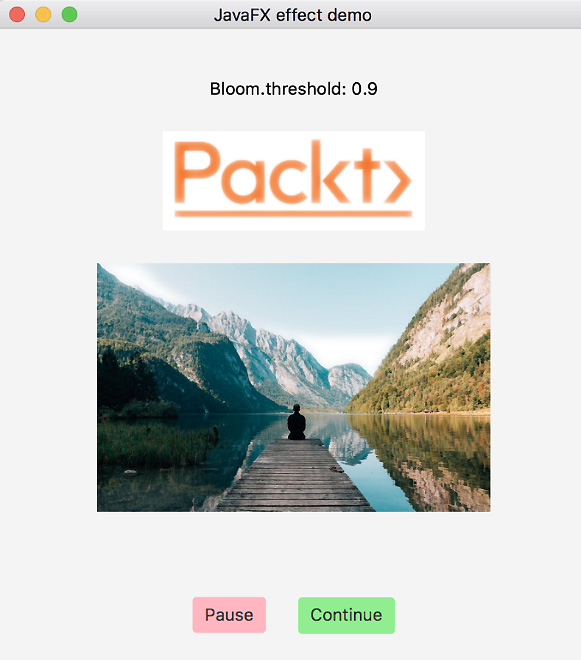``//double d = 0.9;``
``txt.setText(effect + ".threshold: " + d);``
``Bloom b = new Bloom();``
``b.setThreshold(d);``
• 参数值2的效果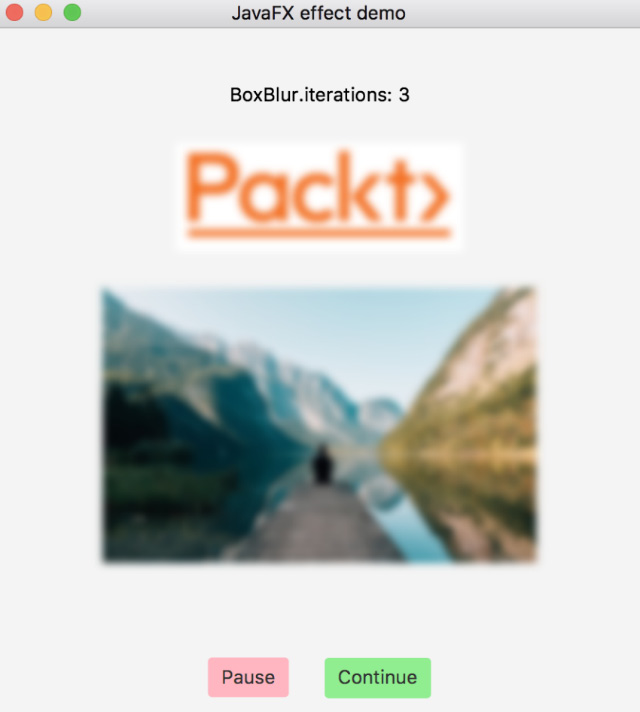``// double d = 0.3;``
``int i = (int) d * 10;``
``int it = i / 3;``
``txt.setText(effect + ".iterations: " + it);``
``BoxBlur bb = new BoxBlur();``
``bb.setIterations(i);``
• 参数值3的效果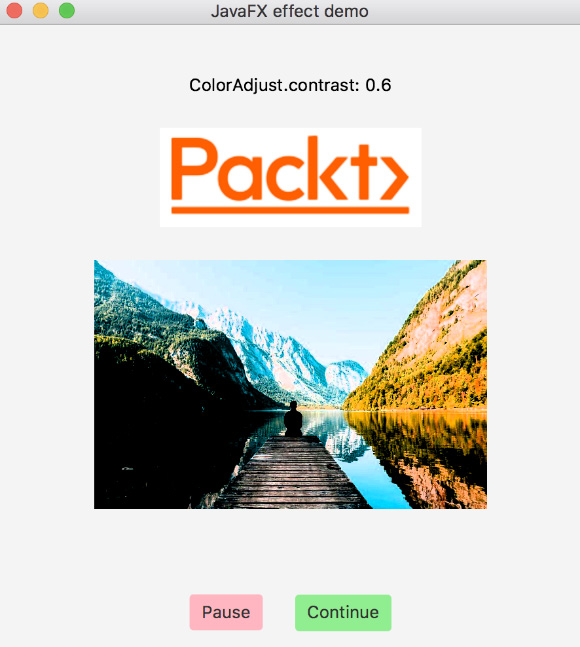``double c = Math.round((-1.0 + d * 2) * 10.0) / 10.0;     // 0.6``
``txt.setText(effect + ": " + c);``
``ColorAdjust ca = new ColorAdjust();``
``ca.setContrast(c);``
• 参数值4的效果：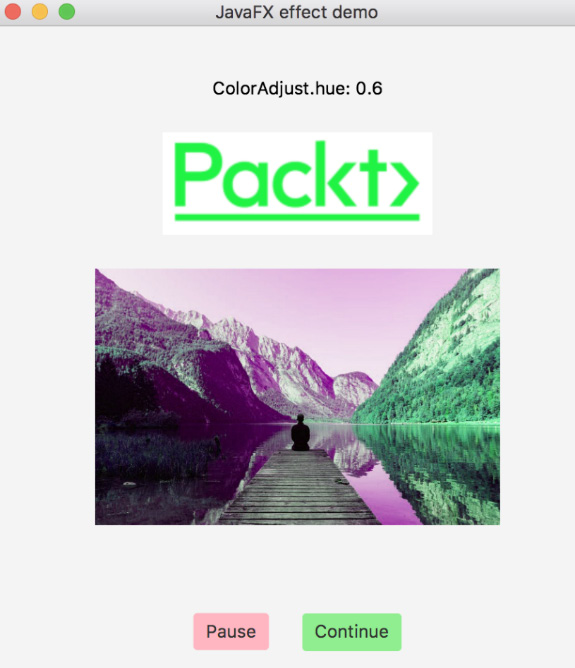``double h = Math.round((-1.0 + d * 2) * 10.0) / 10.0;     // 0.6``
``txt.setText(effect + ": " + h);``
``ColorAdjust ca1 = new ColorAdjust();``
``ca1.setHue(h);``
• 参数值5的效果：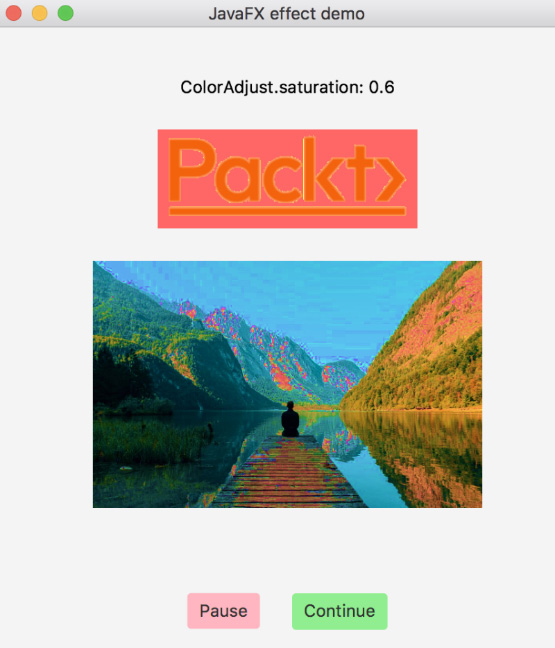``double st = Math.round((-1.0 + d * 2) * 10.0) / 10.0;    // 0.6``
``txt.setText(effect + ": " + st);``
``ColorAdjust ca3 = new ColorAdjust();``
``ca3.setSaturation(st);``
• 参数值6的效果：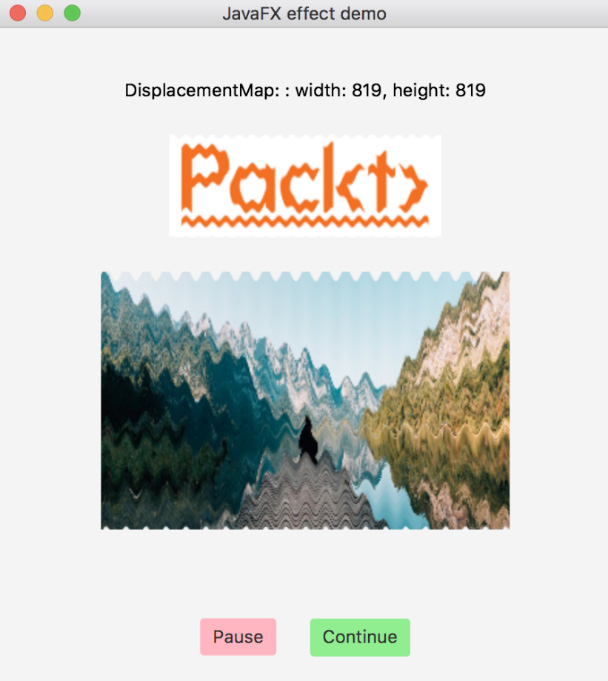``int w = (int)Math.round(4096 * d);  //819``
``int h1 = (int)Math.round(4096 * d); //819``
``txt.setText(effect + ": " + ": width: " + w + ", height: " + ``
``                                                           h1);``
``DisplacementMap dm = new DisplacementMap();``
``FloatMap floatMap = new FloatMap();``
``floatMap.setWidth(w);``
``floatMap.setHeight(h1);``
``for (int k = 0; k < w; k++) {``
``    double v = (Math.sin(k / 20.0 * Math.PI) - 0.5) / 40.0;``
``    for (int j = 0; j < h1; j++) {``
``        floatMap.setSamples(k, j, 0.0f, (float) v);``
``    }``
``}``
``dm.setMapData(floatMap);``
• 参数值 7 的影响：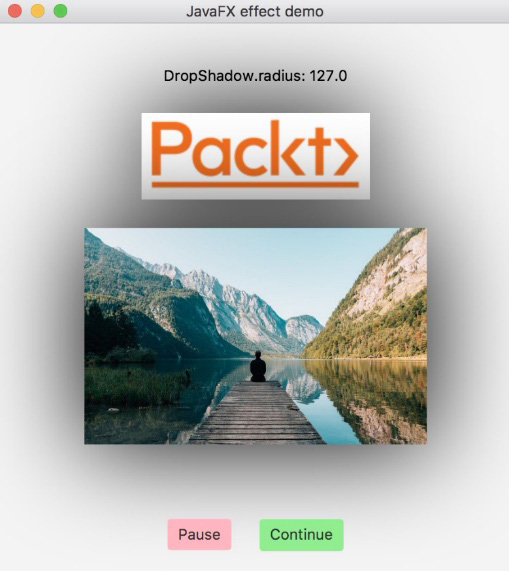``double rd = Math.round((127.0 * d) * 10.0) / 10.0; // 127.0``
``System.out.println(effect + ": " + rd);``
``txt.setText(effect + ": " + rd);``
``DropShadow sh = new DropShadow();``
``sh.setRadius(rd);``
• 参数值8的效果：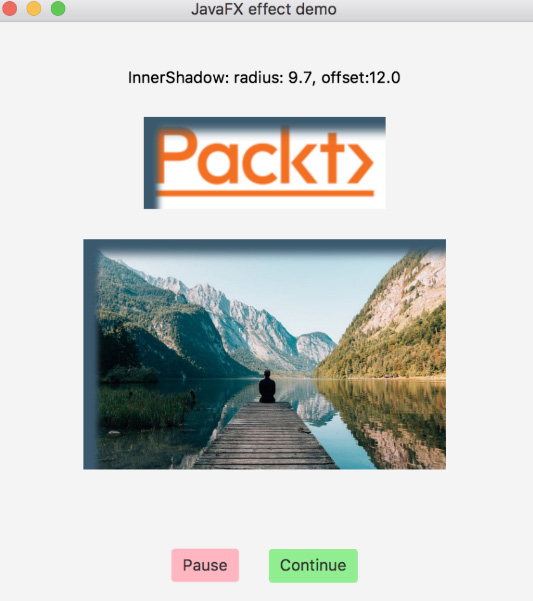``double rad = Math.round(12.1 * d *10.0)/10.0;      // 9.7``
``double off = Math.round(15.0 * d *10.0)/10.0;      // 12.0``
``txt.setText("InnerShadow: radius: " + rad + ", offset:" + off);``
``InnerShadow is = new InnerShadow();``
``is.setColor(Color.web("0x3b596d"));``
``is.setOffsetX(off);``
``is.setOffsetY(off);``
``is.setRadius(rad);``
• 参数值9的效果：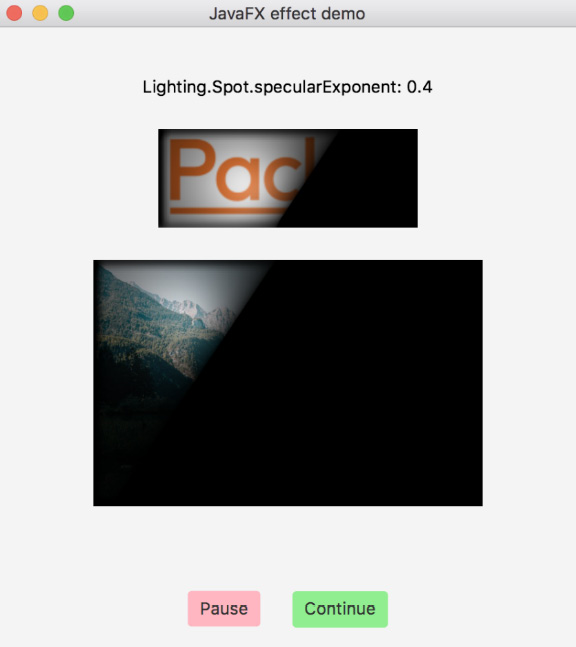``double sS = Math.round((d * 4)*10.0)/10.0;      // 0.4``
``txt.setText(effect + ": " + sS);``
``Light.Spot lightSs = new Light.Spot();``
``lightSs.setX(150);``
``lightSs.setY(100);``
``lightSs.setZ(80);``
``lightSs.setPointsAtX(0);``
``lightSs.setPointsAtY(0);``
``lightSs.setPointsAtZ(-50);``
``lightSs.setSpecularExponent(sS);``
``Lighting lSs = new Lighting();``
``lSs.setLight(lightSs);``
``lSs.setSurfaceScale(5.0);``
• 参数值10的效果：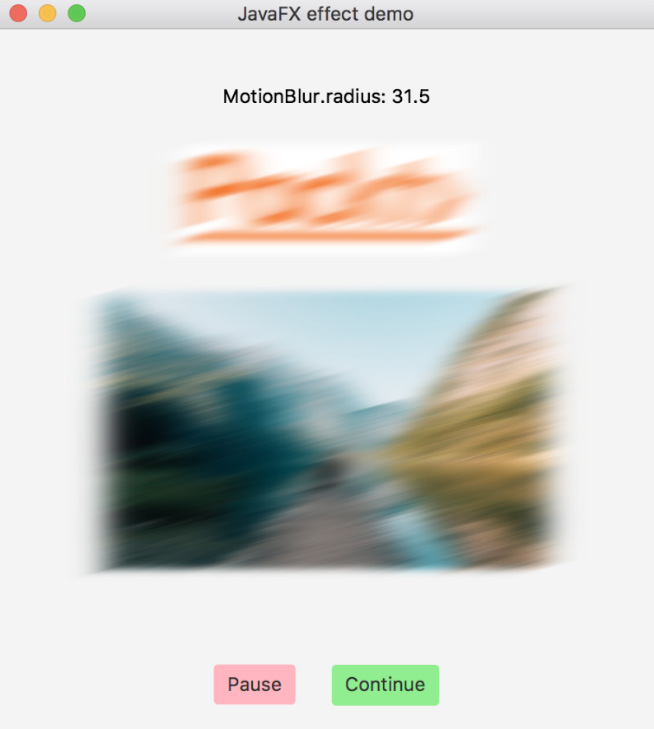``double r = Math.round((63.0 * d)*10.0) / 10.0;      // 31.5``
``txt.setText(effect + ": " + r);``
``MotionBlur mb1 = new MotionBlur();``
``mb1.setRadius(r);``
``mb1.setAngle(-15);``
• 参数值11的效果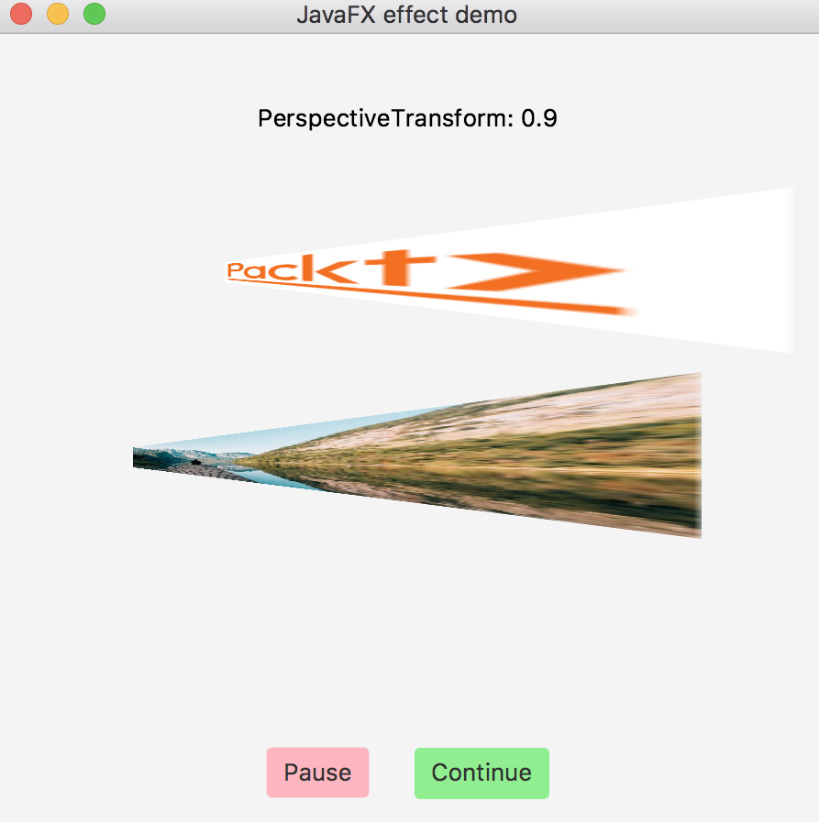``// double d = 0.9;``
``txt.setText(effect + ": " + d); ``
``PerspectiveTransform pt =``
``        new PerspectiveTransform(0., 1. + 50.*d, 310., 50. - ``
``       50.*d, 310., 50. + 50.*d + 1., 0., 100. - 50. * d + 2.);``
• 参数值12的效果：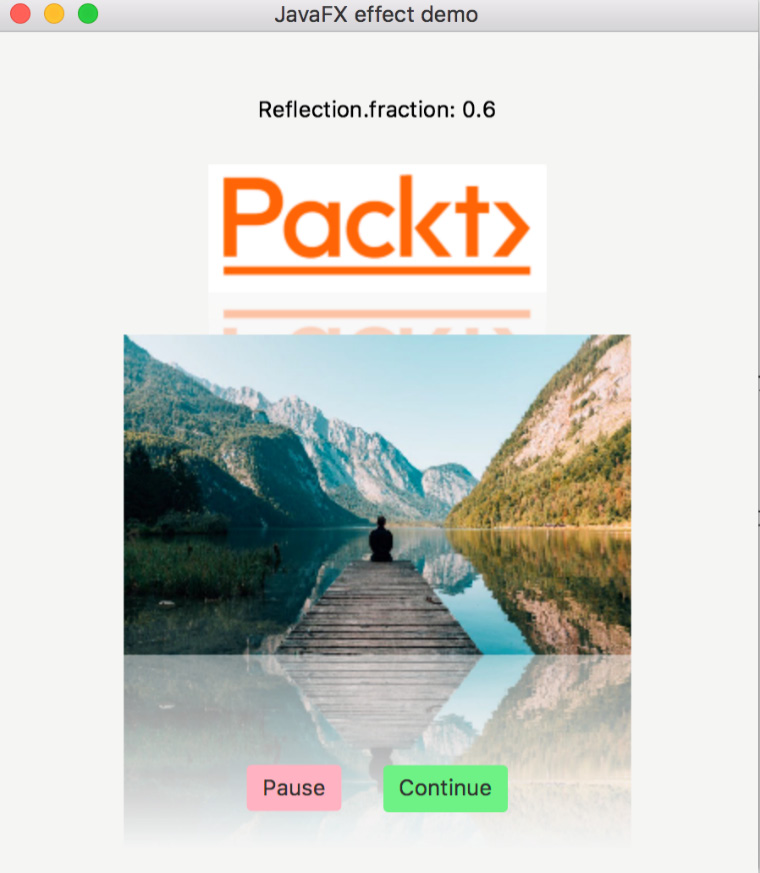``// double d = 0.6;``
``txt.setText(effect + ": " + d);``
``Reflection ref = new Reflection();``
``ref.setFraction(d);``
• 参数值13的效果：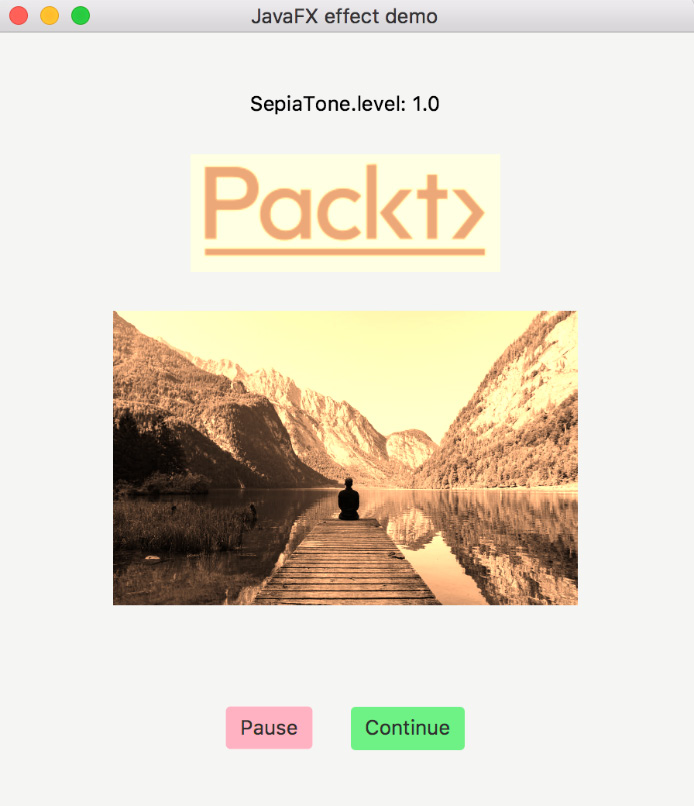``// double d = 1.0;``
``txt.setText(effect + ": " + d);``
``SepiaTone sep = new SepiaTone();``
``sep.setLevel(d);``

# Quiz

1. JavaFX 中的顶级内容容器是什么？
2. JavaFX 中所有场景参与者的基类是什么？
3. 命名 JavaFX 应用程序的基类。
4. JavaFX 应用程序必须实现的一种方法是什么？
5. `Application` 方法必须由 `main` 方法调用才能执行 JavaFX 应用程序？
6. 执行 JavaFX 应用程序需要哪两个 VM 选项？
7. 使用右上角的 x 按钮关闭 JavaFX 应用程序窗口时会调用哪个 `Application` 方法？
8. 必须使用哪个类来嵌入 HTML？
9. 说出三个必须用来播放媒体的类。
10. 播放媒体需要添加什么 VM 选项？
11. 说出五种 JavaFX 效果。